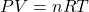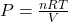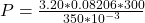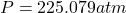## A 3.20-mol sample of gas occupies a volume of 350. mL at 300.0 K. Determine the pressure of the gas.

Question

A 3.20-mol sample of gas occupies a volume of 350. mL at 300.0 K. Determine the

pressure of the gas.

in progress 0
5 months 2021-08-15T15:46:49+00:00 1 Answers 23 views 0

## Answers ( )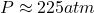Explanation:

From the question we are told that:

Moles of sample n=3.20_mol

Volume V=350mL

Temperature T=300k

Generally the equation for ideal gas is mathematically given by